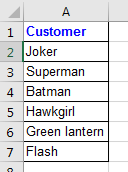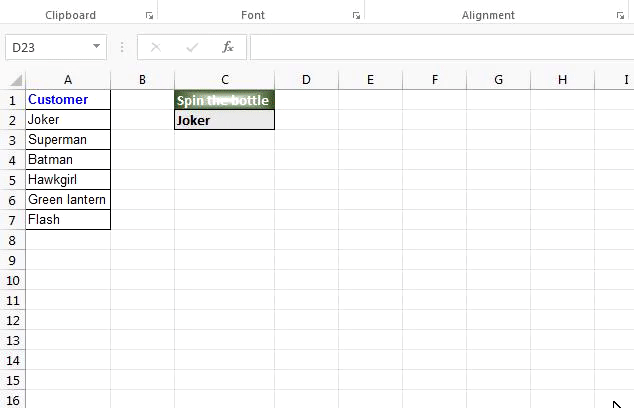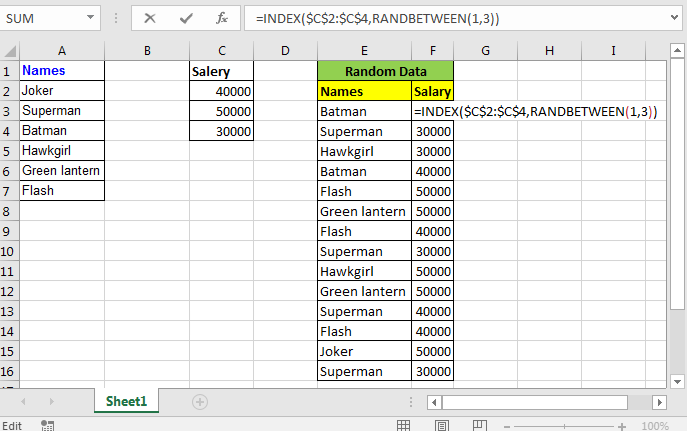# Excel Random Selection: How to Get Random Sample From a Dataset

To randomise a List in excel we will use INDEX function and RANDBETWEEN function. Let's see how we can randomize names in excel. We can randomise not only the names but list of anything.

### Generic Formula

=INDEX(list,RANDBETWEEN(1,count of items in list)

INDEX returns value in a list at the given index. You can learn more about INDEX function here

RANDBETWEEN gives random integers between two specified values. You can learn more about the RANDBETWEEN function here.

Let's have a “random” example.

### Random Name Chooser - Example 1

Let's assume that you and your friends want to play 'spin the bottle game' but you are in the office. You can’t spin a bottle here, right? I can’t. So you want to excel to choose a random name from the names of your friends.

First, prepare the list of names.Now in any cell write this Excel formula

=INDEX(A2:A7,RANDBETWEEN(1,6))

Now whenever you’ll do something in your sheet it will give a random name.### Create Random Data - Example 2

I often need to create random data. So instead of copying I just use the above formula. I just locked the reference to the list.

=INDEX(\$A\$2:\$A\$7,RANDBETWEEN(1,6))

I drag down the formula to the desired position and I get random data to do some practice work.So yeah guys! This is the way you can randomize a list in Excel. Feel free to ask the question in the comments section below.

Related Articles:

Excel Random Selection: How to Get Random Sample From a Dataset

How to Get Random numbers between two numbers

How to Use RAND Function in Excel

Excel RANDBETWEEN Function

Get Random number From Fixed Options

How to Generate Random Phone Numbers in Excel

How to Generate Random values from a list in Excel

Popular Articles:

50 Excel Shortcuts to Increase Your Productivity

How to use the VLOOKUP Function in Excel

How to use the COUNTIF function in Excel

How to use the SUMIF Function in Excel

Terms and Conditions of use

The applications/code on this site are distributed as is and without warranties or liability. In no event shall the owner of the copyrights, or the authors of the applications/code be liable for any loss of profit, any problems or any damage resulting from the use or evaluation of the applications/code.